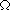HOME TOP UP PREV NEXT 1 GERMAN MAP      Tractatus Logico-Philosophicus 6.24

6.24
The method by which mathematics arrives at its equations is the method of substitution.

For equations express the substitutability of two expressions, and we proceed from a number of equations to new equations, replacing expressions by others in accordance with the equations.

6.241
Thus the proof of the proposition 2æ2=4 runs:
(v)ç'x=væç'x Def.2æ2'x = (2)2'x = (2)1+1'x =2'2'x =1+1'1+1'x
= (')'(')'x =''''x =1+1+1+1'x =4'x.

HOME TOP UP PREV NEXT 1 GERMAN MAP      Tractatus Logico-Philosophicus 6.24